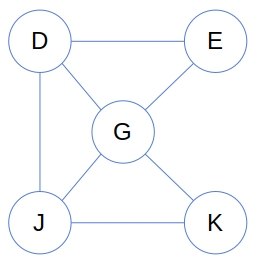# What is an induced subgraph? Explain its types with examples.

An induced subgraph is a special case of a subgraph. If S is a subset of G‘s nodes, then the subgraph of G induced by S is the graph that has S as its set of vertices and contains all the edges of G that have both endpoints in S. This definition covers both directed and undirected graphs. Also, it covers the weighted ones just as the unweighted ones.

Given G and S, the induced subgraph is unique. There’s only one subgraph induced by {D, E, G, J, K} in the above graph:Types of Induced Sub-Graph:

1) Vertex-Induced Sub-Graph:

2) Edge Induced Sub-Graph: